LTE Advanced - PUCCH                            Home : www.sharetechnote.com

When you are thinking of LTE Advanced, the first feature you would think would be `Carrier Aggregation`. And when you think of Carrier Aggregation, the first question you may ask would be "If a UE is getting data from multiple carriers, how can it report ACK/NACK ?"

With this question in mind,you may easily guess that we may need some additional way to handle this situation and this is main motivation of having additional PUCCH format as shown below. Just compare the following two specs, you would notice that we have longer list in Release 10. (Refer to 36.213, 10.1.1 PUCCH format information)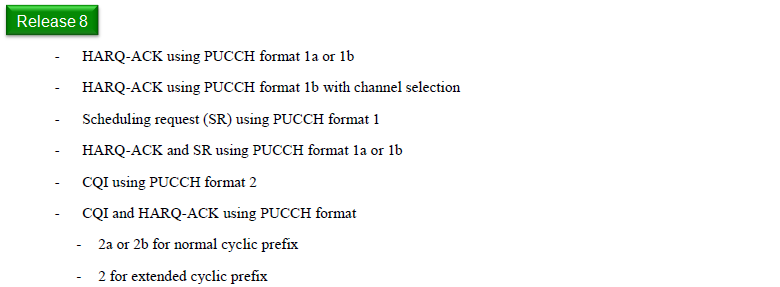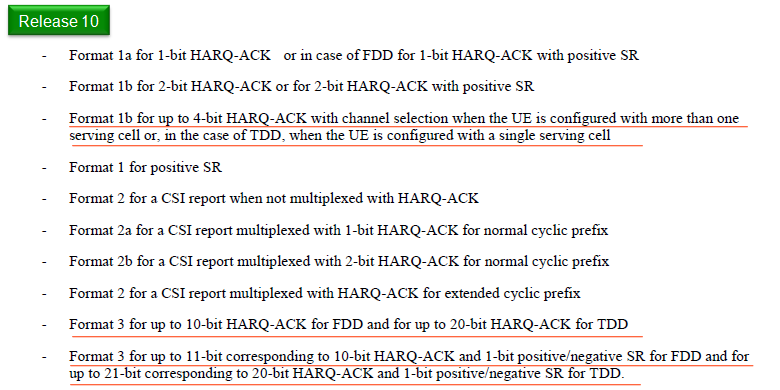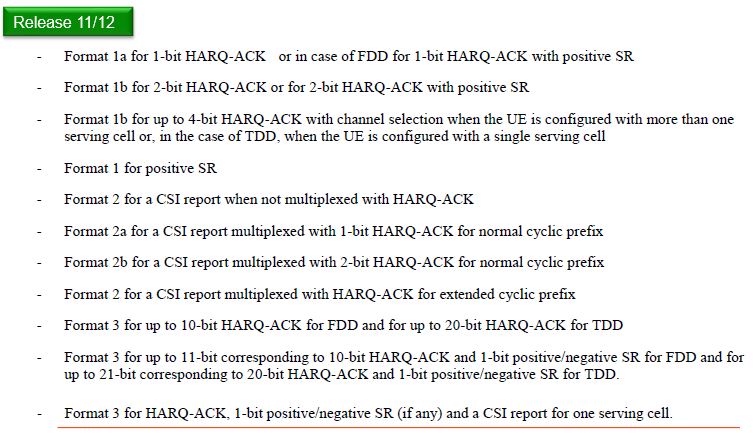Now let's think again more seriously on the question "If a UE is getting data from multiple carriers, how can it report ACK/NACK ?"

If you are a designer, how do you handle this ? I think anybody might think of following options.

• Option 1 : Let UE send 'ACK' only when it was successful to decode PDSCH from all component carriers and transmit NACK if any of PDSCH is failed to be decoded. --> This is technically possible, you would clearly see this would be very inefficient way and cause a lot of unnecessary retransmission. So this method is not considered in real implementation.
• Option 2 : Let UE send ACK or NACK for each carrier separately. --> This would not sound perfect way, but we can reuse the existing PUCCH format without much modification.
• Option 3 : Let UE send ACK or NACK for each carrier on a single PUCCH. --> This would sound the best, but you can easily guess we would need a new PUCCH format since all the existing PUCCH format (Rel 8 format) is designed to send ACK/NAC for one carrier.

In real implementation (specification), Option 2 and 3 are adopted. Two new PUCCH types are introduced for Carrier Aggregation. 'PUCCH format 1b with channel selection' and PUCCH format 3 are those new types.

'PUCCH format 1b with channel selection' can support maximum 2 Carriers only and PUCCH format 3 can support up to 5 carriers in release 11 and 12 and support up to 10 carriers in release 13 and 14.

Followings are the list of topics that will be described in further details in this page.

Format 1b (with Channel Selection)

Format 1b is not a new one. It was there for LTE release 8, but it has been extended to send 4 bit HARQ-ACK/NACK in LTE advanced.

In this section, you would see one of the most complicated and confusing tables in the whole LTE specification (at least to me, it was the most confusing one). The interpretation of the table is even more confusing.

My first question was why they designed ACK/NACK transmission mechanism in such a akward/confusing manner ?

Of course, 3GPP specification never gives any explicit answer to this kind of question. With a lot of thinking and talking to other expert engineers, I personally came to the conclusion that we need to use this kind of strange way because PUCCH 1b can carry only two bits of data, which means it can carry only 4 different state information. But the number of possible ACK/NACK/DTX combination for two carriers is much larger than that. In case of 2 CC MIMO, there can be 81 different combinations. Definately it is impossible to handle this kind of combination with only 2 bit information. Due to this bit size restriction, they decided to use PUCCH position as a kind of additional status bits. We use N1PUCCH for this purpose and we can have 4 different values for this parameter.

Getting confused already ?

I fully understand how you feel about this. Just pass on and see if you have clearer understanding after you go through the specific examples in this page.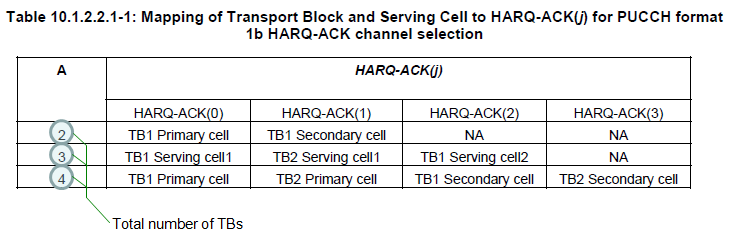< When A = 2 >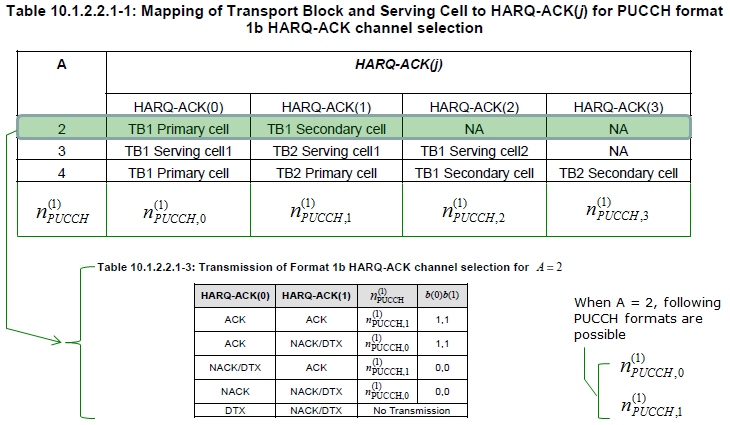Note : What is meaning of NACK/DTX ?

It means NACK or DTX. For example, in case of fourth item.. Network can determine that UE has send explicit 'NACK' for PDSCH on PCC, but it cannot determine whether UE has sent explicit NACK or does not sent any NACK nor ACK for SCC.

Then you would ask why we use this kind of ambiguous expression ? It may be to reduce the number of combination. If we need to handle NACK and DTX separately in every cases, the number of raws (combination) in the above table should be longer. In that case, we may need more information bits which can cause additional overhead.

To make it even more complicated, you need to take into account the following statement as well to fully interpret the table. (Following statement is from 36.213 10.1.2.2 FDD HARQ-ACK procedures for more than one configured serving cell)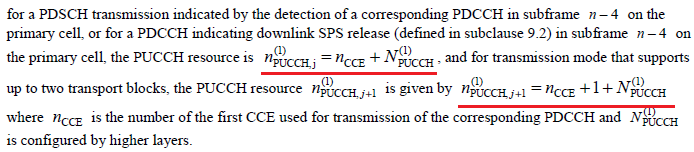To understand the meaning of this table more clearly, let me give you an example case.

Let assume

i)  sib2.radioResourceConfigCommon.pucch-ConfigCommon.n1PUCCH-AN = 0

ii) TPC Command for PUCCH = 00

iii)I am applying following case.< Case 1 >

If HARQ-ACK(0) = ACK and HARQ-ACK(1) = ACK,

UE report (b(0)b(1) = (1,1)) via n1PUCCH= N1PUCCH-AN-CS-r10 = 10

< Case 2>

If HARQ-ACK(0) = ACK and HARQ-ACK(1) = NACK/DTX,

UE report (b(0)b(1) = (1,1)) via n1PUCCH =  n1PUCCH-AN = (0 + n_CCE).

< Case 3>

If HARQ-ACK(0) = NACK/DTX and HARQ-ACK(1) = ACK,

UE report (b(0)b(1) = (0,0)) via n1PUCCH= N1PUCCH-AN-CS-r10 = 10.

< Case 4>

If HARQ-ACK(0) = NACK and HARQ-ACK(1) = NACK/DTX,

UE report (b(0)b(1) = (0,0)) via n1PUCCH= n1PUCCH-AN = (0 + n_CCE).

One important fact you would notice from this example is as follows :

Even though UE need to notify two PUCCH resources to report HARQ-ACK(0) and HARQ-ACK(1) if it wants to send it separately, by this way it can report  about HARQ-ACK(0) and HARQ-ACK(1) using only one PUCCH resource

Example 1 > --------------------------------------------

Now let's see a more practical example as a practice to understand logic described above. Assume we tested in a following condition.

i)  Carrier Aggregation 2 CC - SISO, NO DCI 0(UL Grant) to suppress PUSCH.

ii) sib2.radioResourceConfigCommon.pucch-ConfigCommon.n1PUCCH-AN = 0

iii) n_CCE = 14 (In reality, this would change subframe by subframe, but let's assume this is fixed)

iv) TPC Command for PUCCH = 00

v)I am applying following case.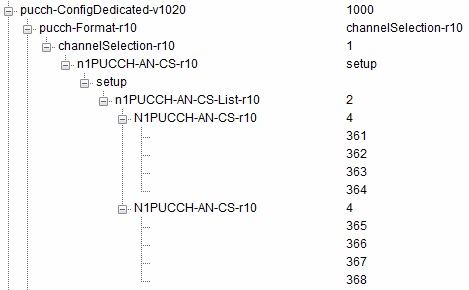If Network received PUCCH as follows. How would interpret the result and fill out the cell (A)~(T)

 Number Timing Recieved PUCCH Interpretation SFN Subframe N1_PUCCH b(0) b(1) HARQ-ACK(0) HARQ-ACK(1) (1) 814 4 361 0 0 (A) (B) (2) 815 2 14 1 1 (C) (D) (3) 694 4 361 0 0 (E) (F) (4) 695 2 14 1 1 (G) (H) (5) 174 4 361 0 0 (I) (J) (6) 175 2 14 1 1 (K) (L) (7) 674 5 361 1 1 (M) (N) (8) 154 4 361 0 0 (O) (P) (9) 155 2 14 1 1 (Q) (R) (10) 658 4 361 0 0 (S) (T)

If you filled out the cells as follows, it implies you understand the logic correctly. If not, read the logic described and try again.

 Number Timing Recieved PUCCH Interpretation SFN Subframe N1_PUCCH b(0) b(1) HARQ-ACK(0) HARQ-ACK(1) 1 814 4 361 0 0 NACK/DTX ACK 2 815 2 14 1 1 ACK NACK/DTX 3 694 4 361 0 0 NACK/DTX ACK 4 695 2 14 1 1 ACK NACK/DTX 5 174 4 361 0 0 NACK/DTX ACK 6 175 2 14 1 1 ACK NACK/DTX 7 674 5 361 1 1 ACK ACK 8 154 4 361 0 0 NACK/DTX ACK 9 155 2 14 1 1 ACK NACK/DTX 10 658 4 361 0 0 NACK/DTX ACK

< When A = 3 >< When A = 4 >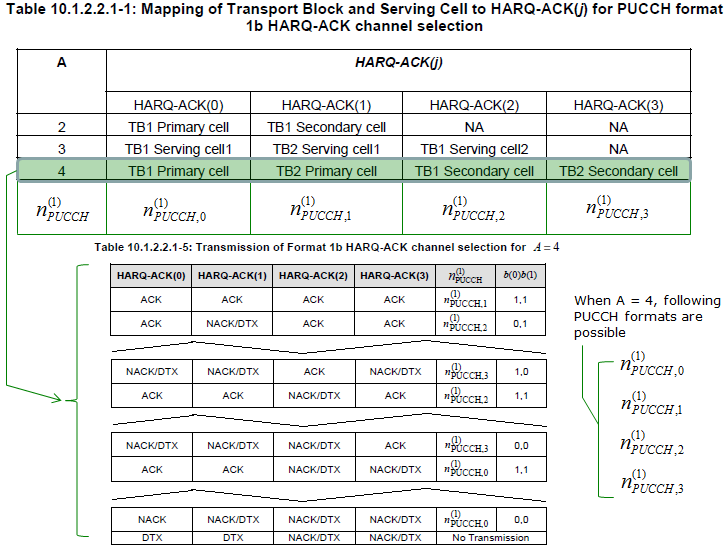To make it even more complicated, you need to take into account the following statement as well to fully interpret the table. (Following statement is from 36.213 10.1.2.2 FDD HARQ-ACK procedures for more than one configured serving cell)To understand the meaning of this table more clearly, let me give you an example case.

Let assume

i)  sib2.radioResourceConfigCommon.pucch-ConfigCommon.n1PUCCH-AN = 0

ii) TPC Command for PUCCH = 00

iii)I am applying following case.< Case 1 >

If HARQ-ACK(0) = ACK and HARQ-ACK(1) = ACK and HARQ-ACK(2) = ACK and HARQ-ACK(3) = ACK,

UE report (b(0)b(1) = (1,1)) via n1PUCCH =  n1PUCCH-AN = (1 + n_CCE)

< Case 2>

If HARQ-ACK(0) = ACK and HARQ-ACK(1) = NACK/DTX and HARQ-ACK(2) = ACK and HARQ-ACK(3) = ACK,

UE report (b(0)b(1) = (0,1)) via n1PUCCH = N1PUCCH-AN-CS-r10 = 10.

< Case 3 >

If HARQ-ACK(0) = NACK/DTX and HARQ-ACK(1) = ACK and HARQ-ACK(2) = ACK and HARQ-ACK(3) = ACK,

UE report (b(0)b(1) = (0,1)) via n1PUCCH =  n1PUCCH-AN = (1 + n_CCE)

< Case 4>

If HARQ-ACK(0) = NACK/DTX and HARQ-ACK(1) = NACK/DTX and HARQ-ACK(2) = ACK and HARQ-ACK(3) = ACK,

UE report (b(0)b(1) = (1,1)) via n1PUCCH = N1PUCCH-AN-CS-r10 = 14.

< Case 5 >

If HARQ-ACK(0) = ACK and HARQ-ACK(1) = ACK and HARQ-ACK(2) = ACK and HARQ-ACK(3) = NACK/DTX,

UE report (b(0)b(1) = (1,0)) via n1PUCCH =  n1PUCCH-AN = (1 + n_CCE)

< Case 6>

If HARQ-ACK(0) = ACK and HARQ-ACK(1) = NACK/DTX and HARQ-ACK(2) = ACK and HARQ-ACK(3) = NACK/DTX,

UE report (b(0)b(1) = (0,0)) via n1PUCCH = N1PUCCH-AN-CS-r10 = 14.

< Case 7 >

If HARQ-ACK(0) = NACK/DTX and HARQ-ACK(1) = ACK and HARQ-ACK(2) = ACK and HARQ-ACK(3) = NACK/DTX,

UE report (b(0)b(1) = (0,0)) via n1PUCCH =  n1PUCCH-AN = (1 + n_CCE)

< Case 8>

If HARQ-ACK(0) = NACK/DTX and HARQ-ACK(1) = NACK/DTX and HARQ-ACK(2) = ACK

and HARQ-ACK(3) = NACK/DTX,

UE report (b(0)b(1) = (1,0)) via n1PUCCH = N1PUCCH-AN-CS-r10 = 14.

< Case 9>

If HARQ-ACK(0) = ACK and HARQ-ACK(1) = ACK and HARQ-ACK(2) = NACK/DTX and HARQ-ACK(3) = ACK,

UE report (b(0)b(1) = (1,1)) via n1PUCCH = N1PUCCH-AN-CS-r10 = 10.

< Case 10>

If HARQ-ACK(0) = ACK and HARQ-ACK(1) = NACK/DTX and HARQ-ACK(2) = NACK/DTX and HARQ-ACK(3) = ACK,

UE report (b(0)b(1) = (1,0)) via n1PUCCH = N1PUCCH-AN-CS-r10 = 10.

< Case 11>

If HARQ-ACK(0) = NACK/DTX and HARQ-ACK(1) = ACK and HARQ-ACK(2) = NACK/DTX and HARQ-ACK(3) = ACK,

UE report (b(0)b(1) = (0,1)) via n1PUCCH = N1PUCCH-AN-CS-r10 = 14.

< Case 12>

If HARQ-ACK(0) = NACK/DTX and HARQ-ACK(1) = NACK/DTX and HARQ-ACK(2) = NACK/DTX

and HARQ-ACK(3) = ACK,

UE report (b(0)b(1) = (0,0)) via n1PUCCH = N1PUCCH-AN-CS-r10 = 14.

< Case 13 >

If HARQ-ACK(0) = ACK and HARQ-ACK(1) = ACK and HARQ-ACK(2) = NACK/DTX and HARQ-ACK(3) = NACK/DTX,

UE report (b(0)b(1) = (1,1)) via n1PUCCH =  n1PUCCH-AN = (0 + n_CCE)

< Case 14 >

If HARQ-ACK(0) = ACK and HARQ-ACK(1) = NACK/DTX and HARQ-ACK(2) = NACK/DTX

and HARQ-ACK(3) = NACK/DTX,

UE report (b(0)b(1) = (1,0)) via n1PUCCH =  n1PUCCH-AN = (0 + n_CCE)

< Case 15 >

If HARQ-ACK(0) = NACK/DTX and HARQ-ACK(1) = ACK and HARQ-ACK(2) = NACK/DTX

and HARQ-ACK(3) = NACK/DTX,

UE report (b(0)b(1) = (0,1)) via n1PUCCH =  n1PUCCH-AN = (0 + n_CCE)

< Case 16 >

If HARQ-ACK(0) = NACK/DTX and HARQ-ACK(1) = NACK and HARQ-ACK(2) = NACK/DTX

and HARQ-ACK(3) = NACK/DTX,

UE report (b(0)b(1) = (0,0)) via n1PUCCH =  n1PUCCH-AN = (0 + n_CCE)

< Case 17 >

If HARQ-ACK(0) = NACK and HARQ-ACK(1) = NACK/DTX and HARQ-ACK(2) = NACK/DTX

and HARQ-ACK(3) = NACK/DTX,

UE report (b(0)b(1) = (0,0)) via n1PUCCH =  n1PUCCH-AN = (0 + n_CCE)

< Case 18 >

If HARQ-ACK(0) = DTX and HARQ-ACK(1) = DTX and HARQ-ACK(2) = NACK/DTX and HARQ-ACK(3) = NACK/DTX,

No Transmission

RRC Message Mapping - Format 1b

Format 1b can be configured in RRC message (RRC Connection Reconfiguration) as shown below.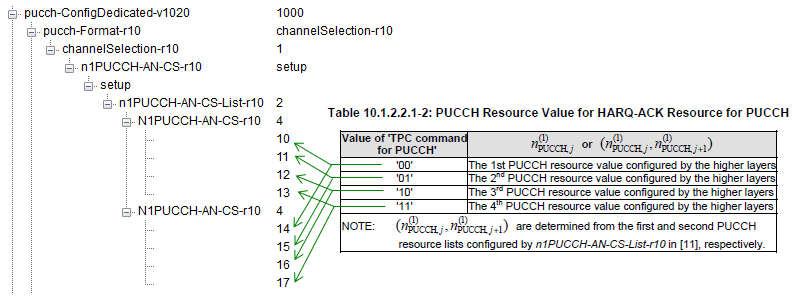Up to 2 CC CA we could handle HARQ Ack/Nack process with Format1b but as you saw in previous section it was super complicated / confusing to understand (I think it is because we have to implement HARQ process for 2 CC with a format which does not have enough number of bits to use. So they needed to use such a strange looking tricks). From 3CC CA, whatever trick you use it would be impossible to handle more than 3CC with format1b, so we need to use PUCCH format which has much larger number of bits in it. It is PUCCH Format 3. However, the way it works is much simpler (at least much simpler to understand). It can have max 11 bits in FDD (21 bits in TDD) for HARQ Ack/Nack that can cover max 5 CC with 2 Codewords and some additional bit for CSI report. In terms of HARQ, it is very simple... just map each bits of the 20 possible bits to each CC (Component Carrier) and each Antenna (if it is MIMO). Overall operation is described as follows in 36.213  10.1.2.2.2 PUCCH format 3 HARQ-ACK procedure.The way you configure PUCCH format 3 in terms of Carrier Aggregation is as follows. Each of the items in n3PUCCH determines the final location of PUCCH on frequency domain at physical subframe (Refer to PUCCH Format 3 page regarding how this parameter determines the physical location of the PUCCH). UE will apply only one parameter of the four values in the RRC message at a specific subframe. Then, how UE can figure out which value to use at each subframe ? It is instructed by eNB via TPC field in DCI as indicated below.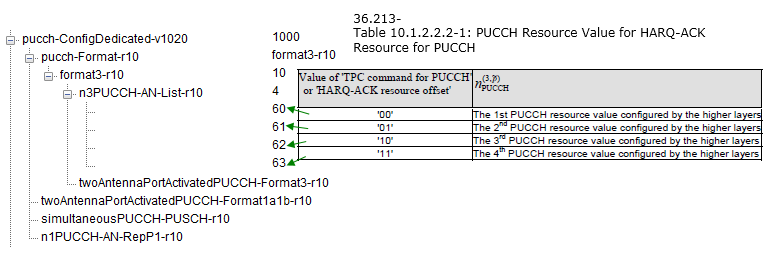Which PUCCH format to use ?

This question is still a challenging one to me. In Carrier Aggregation Condition, it is obvious that we can use PUCCH format1b or PUCCH format 3. However, the tricky thing is to figure out which of the two we need to use.

The most important factors to determine the format is the number of ACK bits required for the specified cell configuration. To understand each and every details of the PUCCH format determination, you would need to understand every single line of following sections in 36.213.

• 10.1.2.2 FDD HARQ-ACK procedures for more than one configured serving cell
• 10.1.3.2 TDD HARQ-ACK procedure for more than one configured serving cell

Following is a summary of format selection based on my experience and the test equipment/UE that I have used. However, I don't think this is the only possible configuration. I will keep adding to the table as I try more.

 Duplex Mode Case PUCCH Format FDD 2CC CA - lower than Category 9 PUCCH Format1b (NOTE 1) 2CC CA - higher than Category 9 PUCCH Format3 (NOTE 2) More than 3CC CA PUCCH Format3 (NOTE 3) TDD 2CC CA (Except Subframe Config 5) PUCCH Format1b (NOTE 4) 2CC CA - Subframe Config 5 PUCCH Format3 (NOTE 5) FDD-TDD 2CC CA - (Primary :FDD, Secondary : TDD) PUCCH Format1b (NOTE 6)

Following is from 36.213 10.1.2.2.

HARQ-ACK transmission on two antenna ports ( p∈[ p0, p1]) is supported for PUCCH format 3.

HARQ-ACK transmission on two antenna ports ( p∈[ p0, p1]) is supported for PUCCH format 1b with channel selection and FDD with two configured serving cells.

Following is from 36.213 10.1.2

For FDD and for a UE transmitting HARQ-ACK using PUCCH format 1b with channel selection or PUCCH format 3, the UE shall determine the number of HARQ-ACK bits, O , based on the number of configured serving cells and the downlink transmission modes configured for each serving cell. The UE shall use two HARQ-ACK bits for a serving cell configured with a downlink transmission mode that support up to two transport blocks; and one HARQ-ACK bit otherwise.

Following is from 36.213 10.1.3.2.2 PUCCH format 3 HARQ-ACK procedure

- for M > 1, and

- for M = 9  <-- M = 9 is for subframe configuration 5. Refer to M definition of LTE-TDD Overview page

the UE shall use PUCCH format 3

NOTE 6 : Refer to 36.213 10.1.2A FDD-TDD HARQ-ACK feedback procedures for primary cell frame structure type 1

A UE that supports aggregating at most 2 serving cells shall use PUCCH format 1b with channel selection for transmission of HARQ-ACK when configured with primary cell frame structure type 1 and secondary cell frame structure type 2.